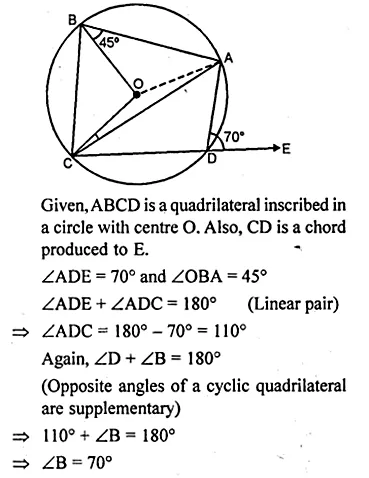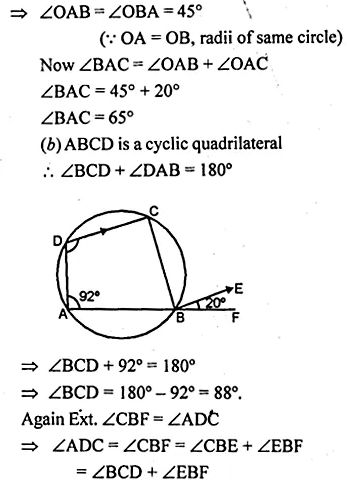Guru

# Question 9. (a) In the figure (i) given below, ABCD is a quadrilateral inscribed in a circle with centre O. CD is produced to E. If ∠ADE = 70° and ∠OBA = 45°, calculate (i) ∠OCA (ii) ∠BAC (b) In figure (ii) given below, ABF is a straight line and BE || DC. If ∠DAB = 92° and ∠EBF = 20°, find : (i) ∠BCD (ii) ∠ADC.

• 0

This is the basic and conceptual question from Chapter name- circles
Topic – Angle properties of circles
Chapter number- 15

In the figure (i)  ABCD is a quadrilateral inscribed in a circle with centre O. CD is produced to E.  ∠ADE = 70° and ∠OBA = 45°, then we have to calculate (i) ∠OCA (ii) ∠BAC

In figure (ii) given below, ABF is a straight line and BE || DC.

∠DAB = 92° and ∠EBF = 20°, now we have to find : (i) ∠BCD (ii) ∠ADC.

ICSE Avichal publication
Understanding ICSE Mathematics
Question 9

Share